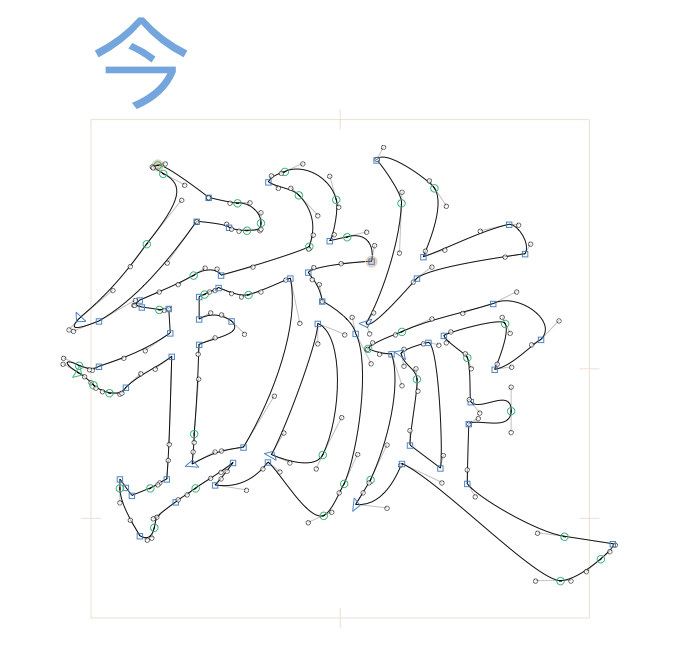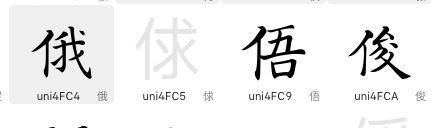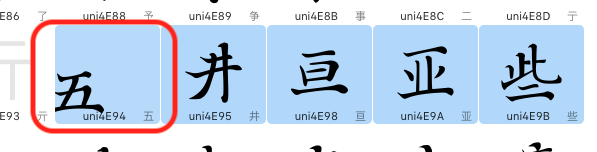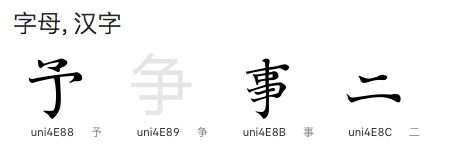# Is there a way to scale the glyphs to certain percentage of the default editing screen

By default the editing view is 1000x1000px, I’m wondering whether there is a plugin or some way to change the glyph size to certain percentage of the default editing screen until horizontally or vertically fitted, and in batch.
Now, the transformation palette can only scale the glyphs to certain percentage of its original sizes.

For example, in the pic below, I want to scale the glyph to 90% of the editing screen (the inner square line). Manual work is exhausting, automatic plugin or script would be very useful.

Final result should be like this:

This script should do what you want:

``````from AppKit import NSAffineTransform, NSMaxY, NSMinX, NSMinY

SCALE_FACTOR = 0.9

for layer in Font.selectedLayers:
bounds = layer.bounds
minY = NSMinY(bounds)
maxY = NSMaxY(bounds)
ascender = layer.ascender
descender = layer.descender
layerHeight = ascender - descender
transform = NSAffineTransform()
transform.scaleBy_(layerHeight / layer.bounds.size.height)
layer.transform(transform)
bounds = layer.bounds
offsetBottom = descender - NSMinY(bounds)
offsetTop = ascender - NSMaxY(bounds)
offset = offsetBottom + offsetTop
transform = NSAffineTransform()
sidebearingCorrection = -NSMinX(bounds) + (layer.width - bounds.size.width) / 2
transform.translateXBy_yBy_(sidebearingCorrection, offsetBottom)
layer.transform(transform)
transform = NSAffineTransform()
transform.translateXBy_yBy_(-layer.width / 2, -layerHeight / 2 - descender)
layer.transform(transform)
transform = NSAffineTransform()
transform.scaleBy_(SCALE_FACTOR)
layer.transform(transform)
transform = NSAffineTransform()
transform.translateXBy_yBy_(layer.width / 2, layerHeight / 2 + descender)
layer.transform(transform)
``````

It scales all selected layers. Paste the code into the Macro panel and run it from there or create a new script so that you can run it from the menu bar or with a keyboard shortcut. Change the value of `SCALE_FACTOR` in the code to scale to a different percentage of the layer height.

you gorgeous.

I tested the script，for many glyphs it works. but for this kind, it did not.
Instead of scaled to 85% I set, it goes far beyond 100%.
I’m afraid you set only one condition “only the height reaches to 85%, and the width will be scaled proportionally” .
The script need to determine which value(width or height) is bigger than the other and then scale the whole glyph to the set percentage proportionally according to the bigger value(width or height).
thanksNew Font.glyphs (9.6 KB)

This should do the trick:

``````from AppKit import NSAffineTransform, NSMaxY, NSMinX, NSMinY

SCALE_FACTOR = 0.9

for layer in Font.selectedLayers:
bounds = layer.bounds
minY = NSMinY(bounds)
maxY = NSMaxY(bounds)
ascender = layer.ascender
descender = layer.descender
layerHeight = ascender - descender
transform = NSAffineTransform()
transform.scaleBy_(layerHeight / layer.bounds.size.height)
layer.transform(transform)
bounds = layer.bounds
offsetBottom = descender - NSMinY(bounds)
offsetTop = ascender - NSMaxY(bounds)
offset = offsetBottom + offsetTop
transform = NSAffineTransform()
sidebearingCorrection = -NSMinX(bounds) + (layer.width - bounds.size.width) / 2
transform.translateXBy_yBy_(sidebearingCorrection, offsetBottom)
layer.transform(transform)
transform = NSAffineTransform()
transform.translateXBy_yBy_(-layer.width / 2, -layerHeight / 2 - descender)
layer.transform(transform)
transform = NSAffineTransform()
scale = SCALE_FACTOR
if bounds.size.width > layer.width:
scale = layer.width * SCALE_FACTOR / bounds.size.width
transform.scaleBy_(scale)
layer.transform(transform)
transform = NSAffineTransform()
transform.translateXBy_yBy_(layer.width / 2, layerHeight / 2 + descender)
layer.transform(transform)
``````

thank you very much

one minor improvement would be perfect.
When there is empty glyph selected, the script will stop working, leave glyphs after the empty unchanged.

For example, I selected 4 (including one empty or more), the last two remain unchanged)Yes, the code assumed that there would be something to scale. I’ve added a check for empty layers:

``````from AppKit import NSAffineTransform
import math

SCALE_FACTOR = 0.9

for layer in Font.selectedLayers:
# get the size and position of the layer contents
bounds = layer.bounds
if bounds.size.width == 0 and bounds.size.height == 0:
# ignore layers without scalable contents
continue
# move center of layer contents to layer origin
transform = NSAffineTransform()
transform.translateXBy_yBy_(-bounds.origin.x - bounds.size.width / 2, -bounds.origin.y - bounds.size.height / 2)
layer.transform(transform)
# find fitting scale by taking the smaller of the fitting width and height scale factors
if bounds.size.width > 0:
scaleWidth = layer.width / bounds.size.width
else:
scaleWidth = math.inf
if bounds.size.height > 0:
scaleHeight = (layer.ascender - layer.descender) / bounds.size.height
else:
scaleHeight = math.inf
fittingScale = min(scaleWidth, scaleHeight) * SCALE_FACTOR
# scale layer contents to desired size
transform = NSAffineTransform()
transform.scaleBy_(fittingScale)
layer.transform(transform)
# move layer contents to the center of the layer
transform = NSAffineTransform()
transform.translateXBy_yBy_(layer.width / 2, layerHeight / 2 + descender)
layer.transform(transform)
``````

this script does work well. I selected 5 glyphs. only the first moved, and it’s not moved to the right position. and if there is empty, it does work either.Do you find something in the macro panel?

I saved it as py file and put into script folder.
The following script works great except when there is empty glyphs selected.

from AppKit import NSAffineTransform, NSMaxY, NSMinX, NSMinY

SCALE_FACTOR = 0.9

for layer in Font.selectedLayers:
bounds = layer.bounds
minY = NSMinY(bounds)
maxY = NSMaxY(bounds)
ascender = layer.ascender
descender = layer.descender
layerHeight = ascender - descender
transform = NSAffineTransform()
transform.scaleBy_(layerHeight / layer.bounds.size.height)
layer.transform(transform)
bounds = layer.bounds
offsetBottom = descender - NSMinY(bounds)
offsetTop = ascender - NSMaxY(bounds)
offset = offsetBottom + offsetTop
transform = NSAffineTransform()
sidebearingCorrection = -NSMinX(bounds) + (layer.width - bounds.size.width) / 2
transform.translateXBy_yBy_(sidebearingCorrection, offsetBottom)
layer.transform(transform)
transform = NSAffineTransform()
transform.translateXBy_yBy_(-layer.width / 2, -layerHeight / 2 - descender)
layer.transform(transform)
transform = NSAffineTransform()
scale = SCALE_FACTOR
if bounds.size.width > layer.width:
scale = layer.width * SCALE_FACTOR / bounds.size.width
transform.scaleBy_(scale)
layer.transform(transform)
transform = NSAffineTransform()
transform.translateXBy_yBy_(layer.width / 2, layerHeight / 2 + descender)
layer.transform(transform)

Can you send me a Glyphs file containing a glyph that does not work? I did rework the code a bit with the latest revision, but that should only have made it more robust :‌|

New Font.glyphs (12.6 KB)Ah, I missed that I still had some variables defined from previous runs in the Macro panel. This fixes that:

``````# Script.final.final.final.py
from AppKit import NSAffineTransform
import math

SCALE_FACTOR = 0.9

for layer in Font.selectedLayers:
# get the size and position of the layer contents
bounds = layer.bounds
if bounds.size.width == 0 and bounds.size.height == 0:
# ignore layers without scalable contents
continue
descender = layer.descender
layerHeight = layer.ascender - descender
# move center of layer contents to layer origin
transform = NSAffineTransform()
transform.translateXBy_yBy_(-bounds.origin.x - bounds.size.width / 2, -bounds.origin.y - bounds.size.height / 2)
layer.transform(transform)
# find fitting scale by taking the smaller of the fitting width and height scale factors
if bounds.size.width > 0:
scaleWidth = layer.width / bounds.size.width
else:
scaleWidth = math.inf
if bounds.size.height > 0:
scaleHeight = layerHeight / bounds.size.height
else:
scaleHeight = math.inf
fittingScale = min(scaleWidth, scaleHeight) * SCALE_FACTOR
# scale layer contents to desired size
transform = NSAffineTransform()
transform.scaleBy_(fittingScale)
layer.transform(transform)
# move layer contents to the center of the layer
transform = NSAffineTransform()
transform.translateXBy_yBy_(layer.width / 2, layerHeight / 2 + descender)
layer.transform(transform)
``````

wow. this is a charm. thank you man, you rock.

I simplified the script a tiny bit. It can be a bit faster, too.

``````# Script.final.final.final.py
from AppKit import NSAffineTransform, NSMidX, NSMidY
import math

SCALE_FACTOR = 0.9

for layer in Font.selectedLayers:
# get the size and position of the layer contents
bounds = layer.bounds
if bounds.size.width < 1 and bounds.size.height < 1:
# ignore layers without scalable contents
continue
descender = layer.descender
layerHeight = layer.ascender - descender
transform = NSAffineTransform()
# find fitting scale by taking the smaller of the fitting width and height scale factors
if bounds.size.width > 0:
scaleWidth = layer.width / bounds.size.width
else:
scaleWidth = math.inf
if bounds.size.height > 0:
scaleHeight = layerHeight / bounds.size.height
else:
scaleHeight = math.inf
fittingScale = min(scaleWidth, scaleHeight) * SCALE_FACTOR

# move center of layer contents to layer origin
transform.shift((-NSMidX(bounds) * fittingScale, -NSMidY(bounds) * fittingScale))
# scale layer contents to desired size
transform.scale(fittingScale)
# move layer contents to the center of the layer
transform.shift((layer.width / 2 / fittingScale, (layerHeight / 2 + descender) / fittingScale))
layer.transform(transform)
``````

thanks.